##### Estimation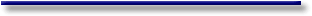# Introduction to Econometrics - Small and large sample properties of estimators

 The property of unbiasedness (for an estimator of theta) is defined by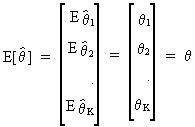(I.VI-1) where the biasvector delta can be written as(I.VI-2) and the precision vector as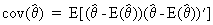(I.VI-3) which is a positive definite symmetric K by K matrix. If two different estimators of the same parameter exist one can compute the difference between their precision vectors: if this vector is positive semi definite this means we know that the second estimator has a "smaller" covariance matrix and can therefore be called better than the first estimator. An estimator is said to be efficient if it is unbiased and at the same the time no other estimator exists with a lower covariance matrix. If Y is a random variable of independent observations with a probability distribution f then the joint distribution can be written as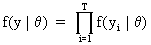(I.VI-4) The function of the unknown parameter, as a function of the values of the random variable, is called the likelihood function which has the same structure as the joint probability function but is dependent on the random variable in stead of the unknown parameter. The information matrix is defined as the negative of the expected value of the Hessian matrix of the log likelihood function L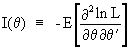(I.VI-5)(I.VI-6) The Cramér-Rao lower bound is defined as the inverse of the information matrix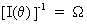(I.VI-7) here denoted omega. If an estimator is unbiased then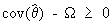(I.VI-8) is a positive semi definite matrix. Expression (I.VI-6) is called the Cramér-Rao inequality. Proof of this inequality can be easily obtained. If we con­sider only one parameter, by definition of the likelihood function we may write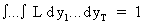(I.VI-9) which can be derived with respect to the parameter(I.VI-10) Deriving a second time yields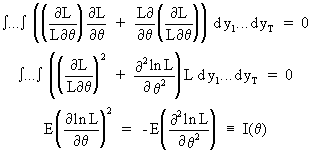(I.VI-11) This implies that E((D ln L)2) = - E(D2 ln L) which is e­quivalent to the information matrix. If the estimator is unbiased then(I.VI-12) It follows from (I.VI-10) that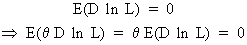(I.VI-13) On combining (I.VI-13) with (I.VI-12) and applying the Cauchy-Schwarz inequality we obtain(I.VI-14) from which the Cramér-Rao inequality follows immediately. Note that according to the Cramér-Rao lower bound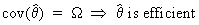(I.VI-15) but not vice versa. This is because the Cramér-Rao lower bound is not always attainable (for unbiased estimators). The property of sufficiency can be formulated as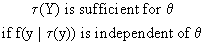(I.VI-16) while the property of consistency is defined as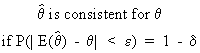(I.VI-17) where delta is a small scalar and epsilon is a vector containing elements with "small" values. The large sample properties apply only when the number of observations converges towards infinity in the limit. Accordingly, we can define the large sample consistency as(I.VI-18) where epsilon is "small". By definition we can also use a shorter notation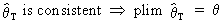(I.VI-19) were "plim" is the so-called "probability limit". In this case we say that the estimator for theta converges in probability to the population value of theta. A short example will clarify the concept of large sample consistency. Let us take the sample mean as an estimator of the population mean. Then it is possible to prove large sample consistency on using eq. (I.III-47) applied to the sample mean: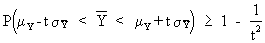(I.VI-20) The standard deviation of the sample mean is known to be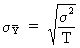(I.VI-21) On combining (I.VI-20) and (I.VI-21) we obtain(I.VI-22)(I.VI-23) Now it obvious that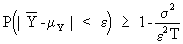(I.VI-24) where the RHS can be made arbitrarily close to 1 by increasing T (the number of sample observations). Now we may conclude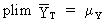(I.VI-25) A sufficient, but not necessary, condition for large sample efficiency is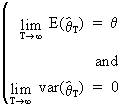(I.VI-26) According to Slutsky's theorem the following holds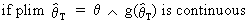(I.VI-27)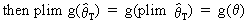(I.VI-28) Other properties of plims are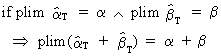(I.VI-29) and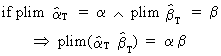(I.VI-30) (this is true even if both estimators are dependent on each other: this is not so with the mathematical expectation) and finally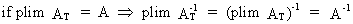(I.VI-31) where AT is a square parameter matrix. Note the following definition of asymptotically distributed parameter vectors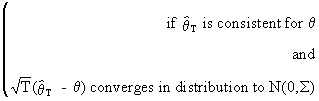(I.VI-32)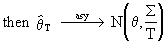(I.VI-33) The concept of asymptotic efficiency can be used to compare estimators. Formally this is written: if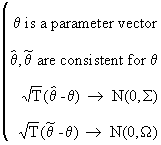(I.VI-34) then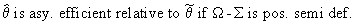(I.VI-35) Finally we describe Cramér's theorem because it enables us to combine plims with convergence in distribution. Formally this theorem states that if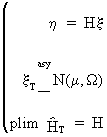(I.VI-36)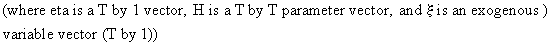then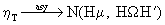(I.VI-37)No news at the moment...© 2000-2018 All rights reserved. All Photographs (jpg files) are the property of Corel Corporation, Microsoft and their licensors. We acquired a non-transferable license to use these pictures in this website.
The free use of the scientific content in this website is granted for non commercial use only. In any case, the source (url) should always be clearly displayed. Under no circumstances are you allowed to reproduce, copy or redistribute the design, layout, or any content of this website (for commercial use) including any materials contained herein without the express written permission.

Information provided on this web site is provided "AS IS" without warranty of any kind, either express or implied, including, without limitation, warranties of merchantability, fitness for a particular purpose, and noninfringement. We use reasonable efforts to include accurate and timely information and periodically updates the information without notice. However, we make no warranties or representations as to the accuracy or completeness of such information, and it assumes no liability or responsibility for errors or omissions in the content of this web site. Your use of this web site is AT YOUR OWN RISK. Under no circumstances and under no legal theory shall we be liable to you or any other person for any direct, indirect, special, incidental, exemplary, or consequential damages arising from your access to, or use of, this web site.

Contributions and Scientific Research: Prof. Dr. E. Borghers, Prof. Dr. P. Wessa
Please, cite this website when used in publications: Xycoon (or Authors), Statistics - Econometrics - Forecasting (Title), Office for Research Development and Education (Publisher), http://www.xycoon.com/ (URL), (access or printout date).